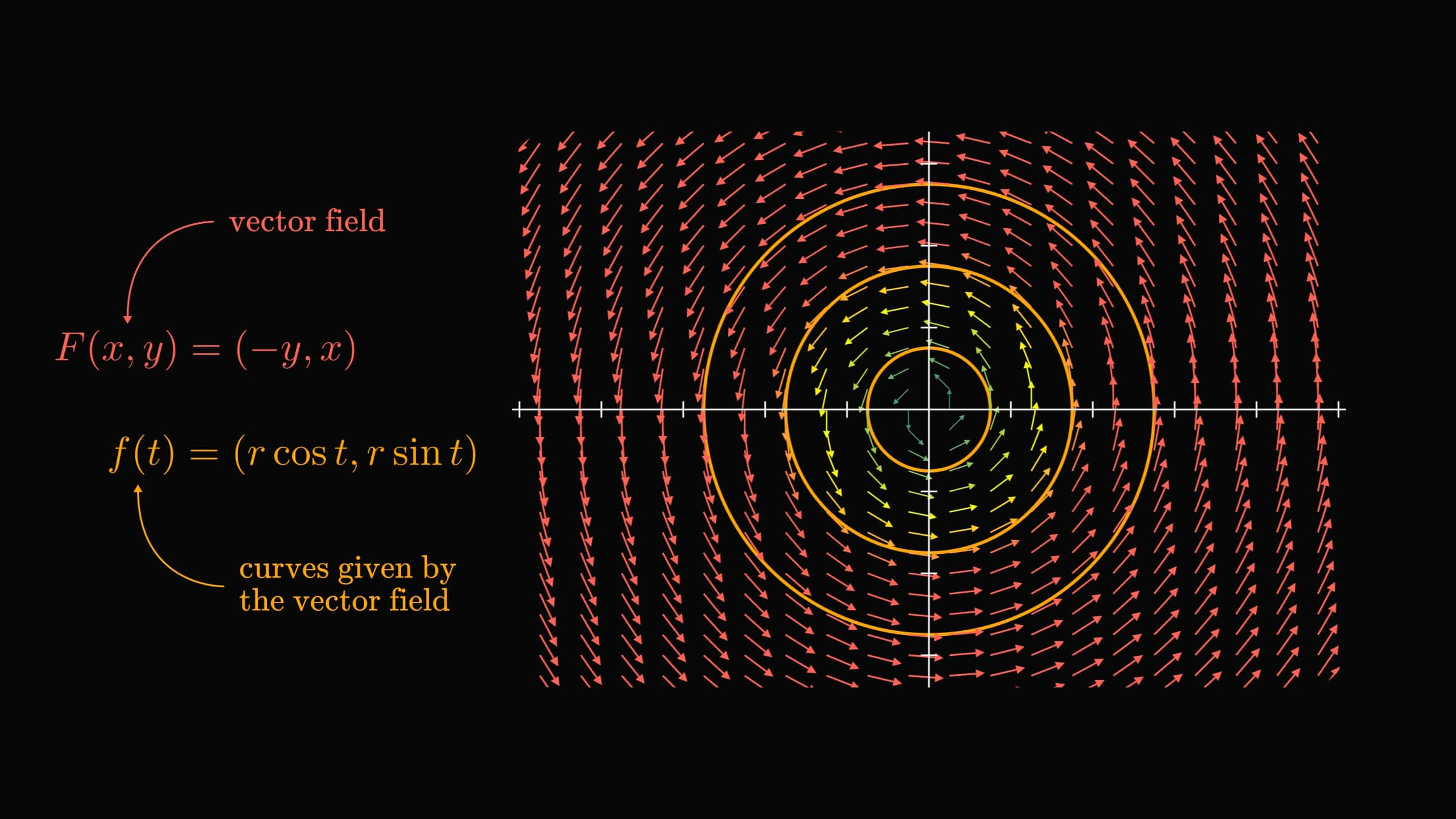# The zoo of multivariable functions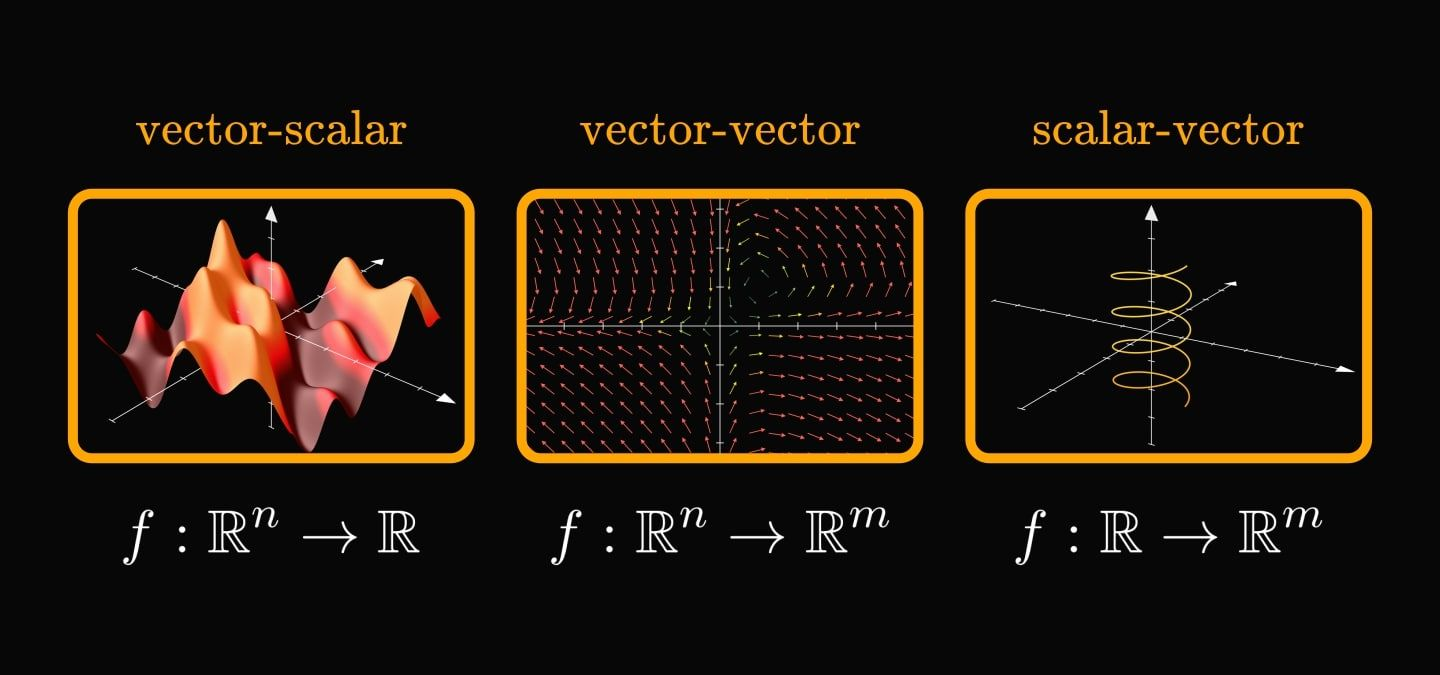In calculus, going from a single variable to millions of variables is hard.

Understanding the three main types of functions helps make sense of multivariable calculus. They all share a deep connection. Let's see why!

In general, a function assigns elements of one set to another.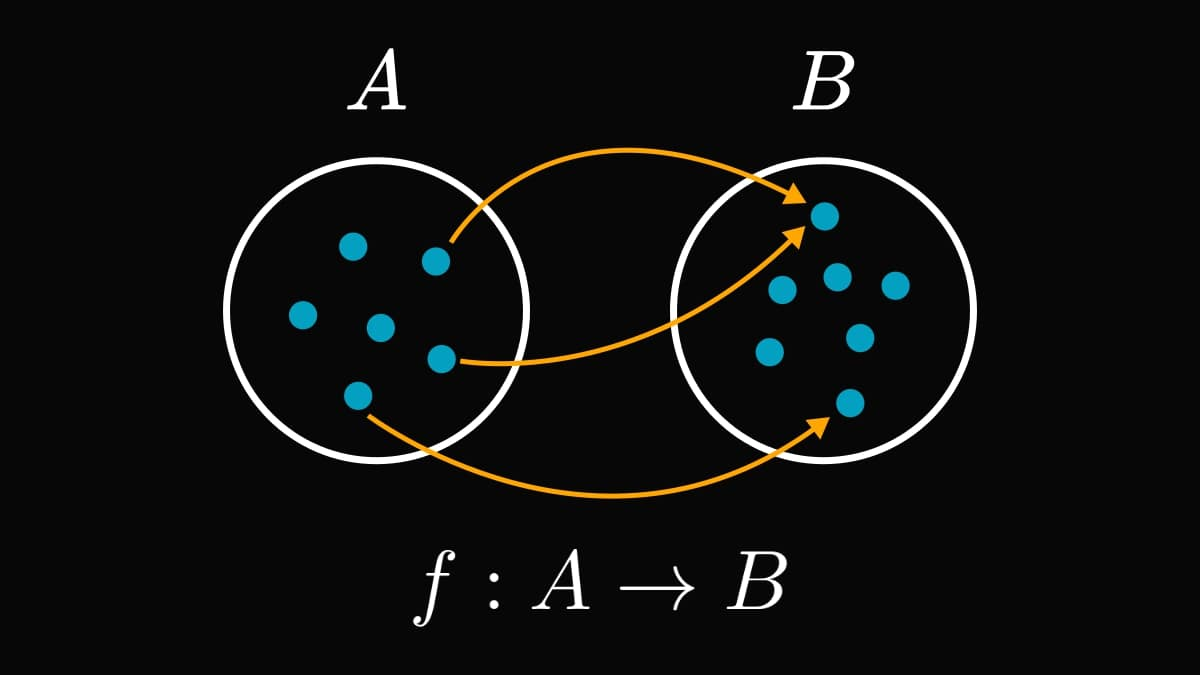This is too abstract for most engineering applications. Let's zoom in a little!

As our measurements are often real numbers, we prefer functions that operate on real vectors or scalars. There are three categories:

1. vector-scalar,
2. vector-vector,
3. and scalar-vector.

When speaking about multivariable calculus, vector-scalar functions come to mind first.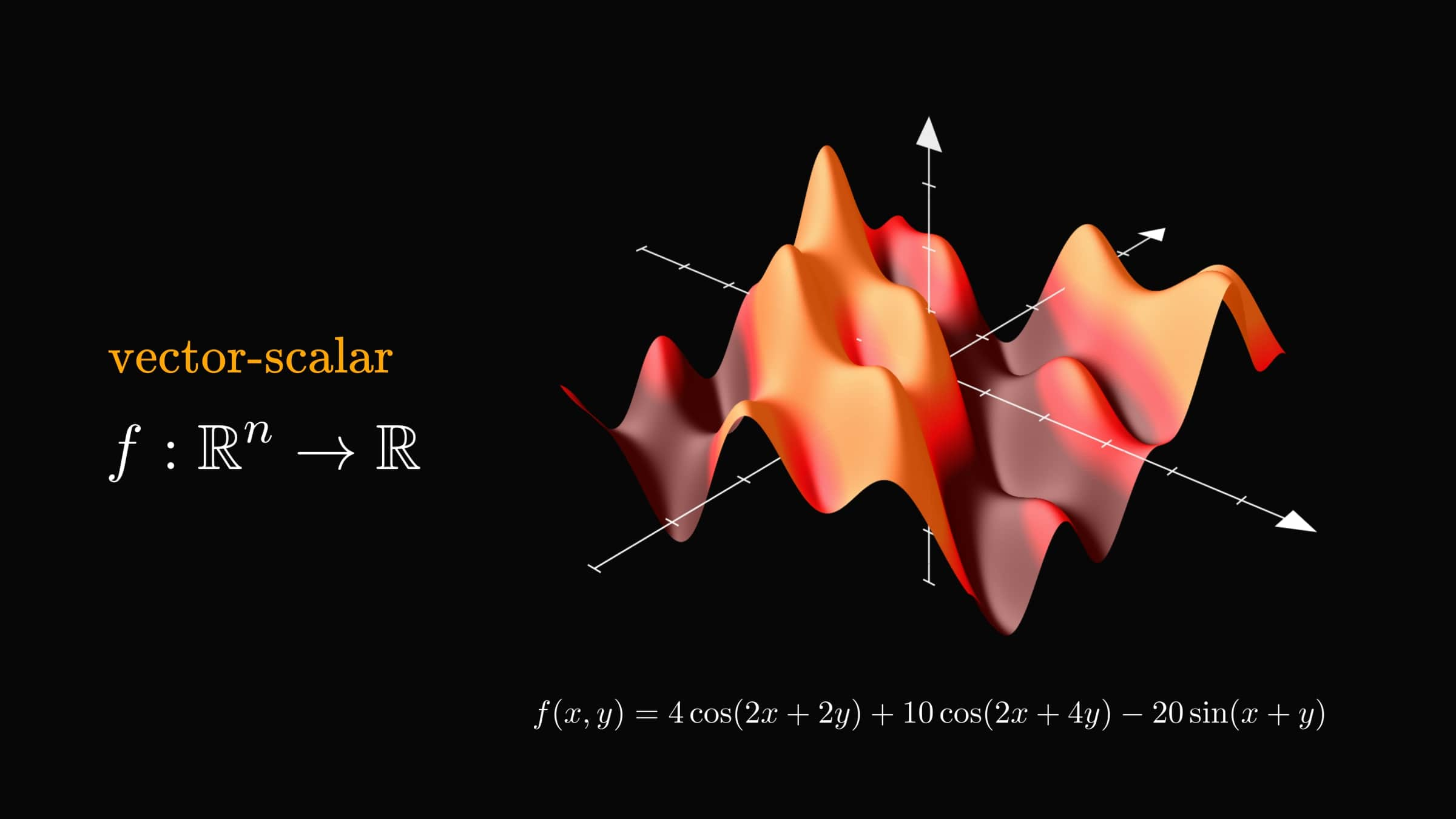Instead of a graph (like their single-variable counterparts), they define surfaces. You can think about a vector-scalar function as a topographic map.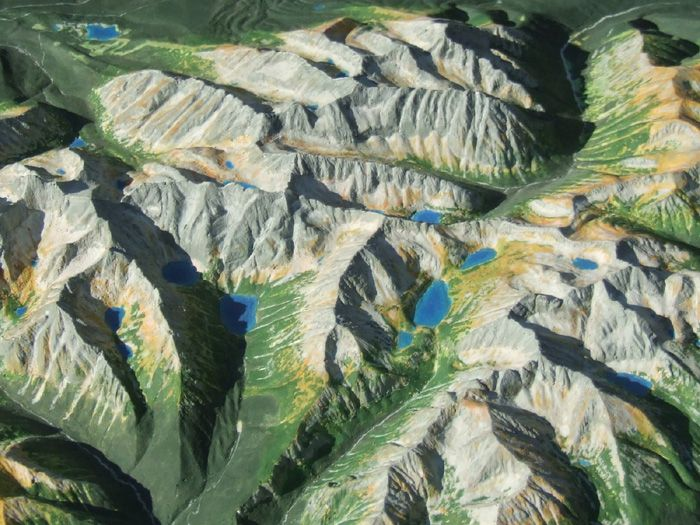(Image source: Wikipedia, https://en.wikipedia.org/wiki/Terrain_cartography)

Although often not denoted, the argument of a vector-scalar function is always a vector. Most frequently, we write out the components - a.k.a. the variables - explicitly.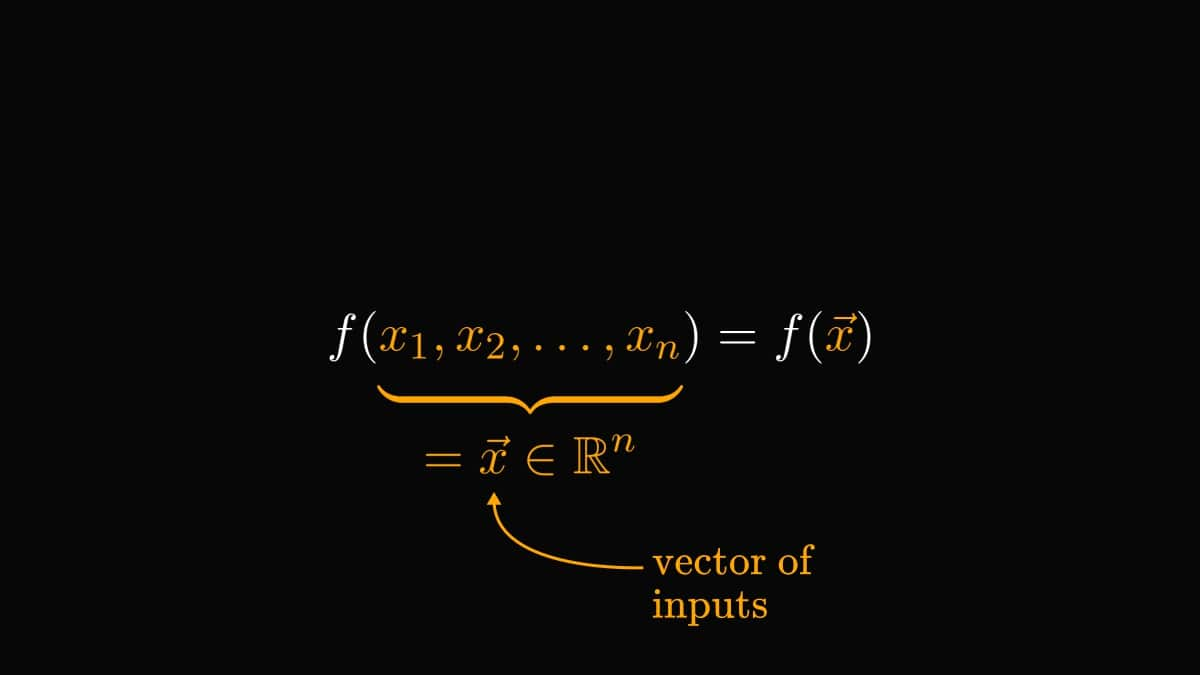Want a practical example of a vector-scalar function? The loss of a predictive model maps the vector of parameters to a single scalar.

Below, you can see the mean-squared error of a simple linear regression model.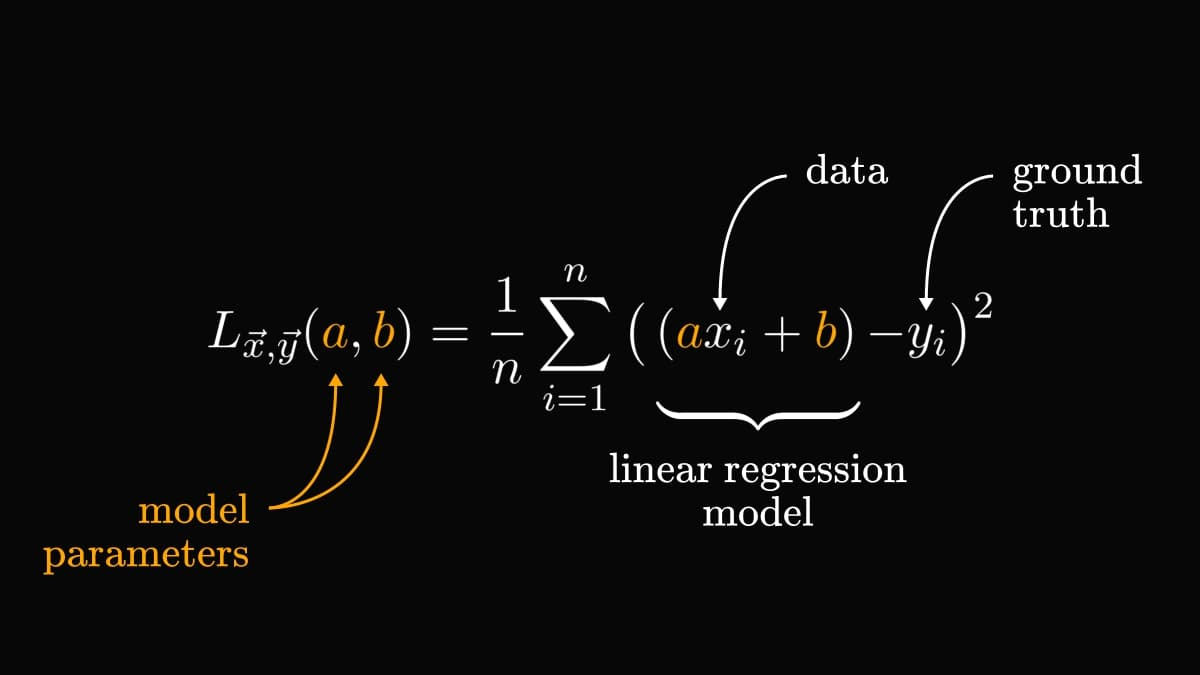Next up, we have the vector-vector functions.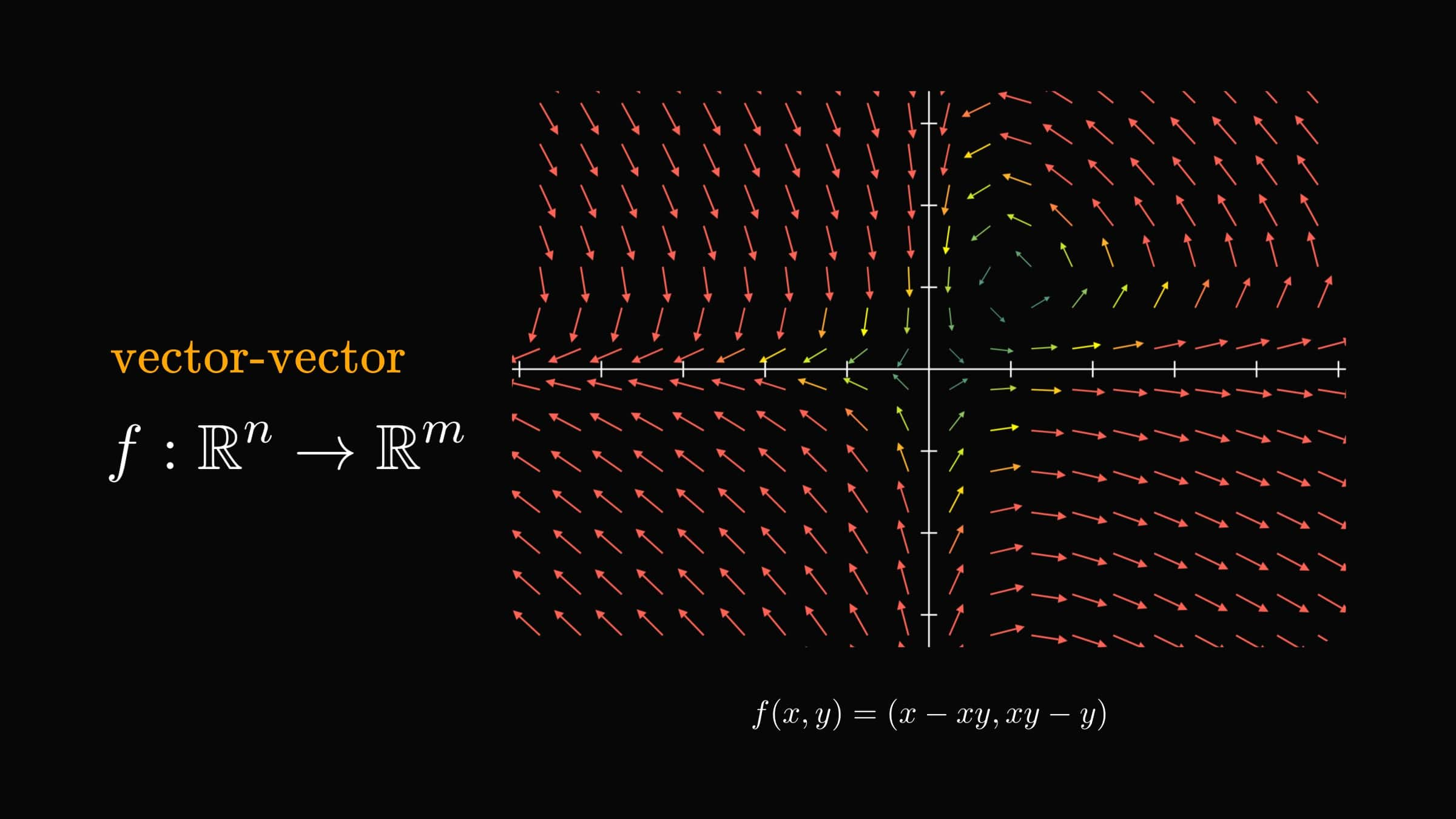You can imagine them as a force field, putting a vector to each point.

The most important example of vector-vector functions is the gradient.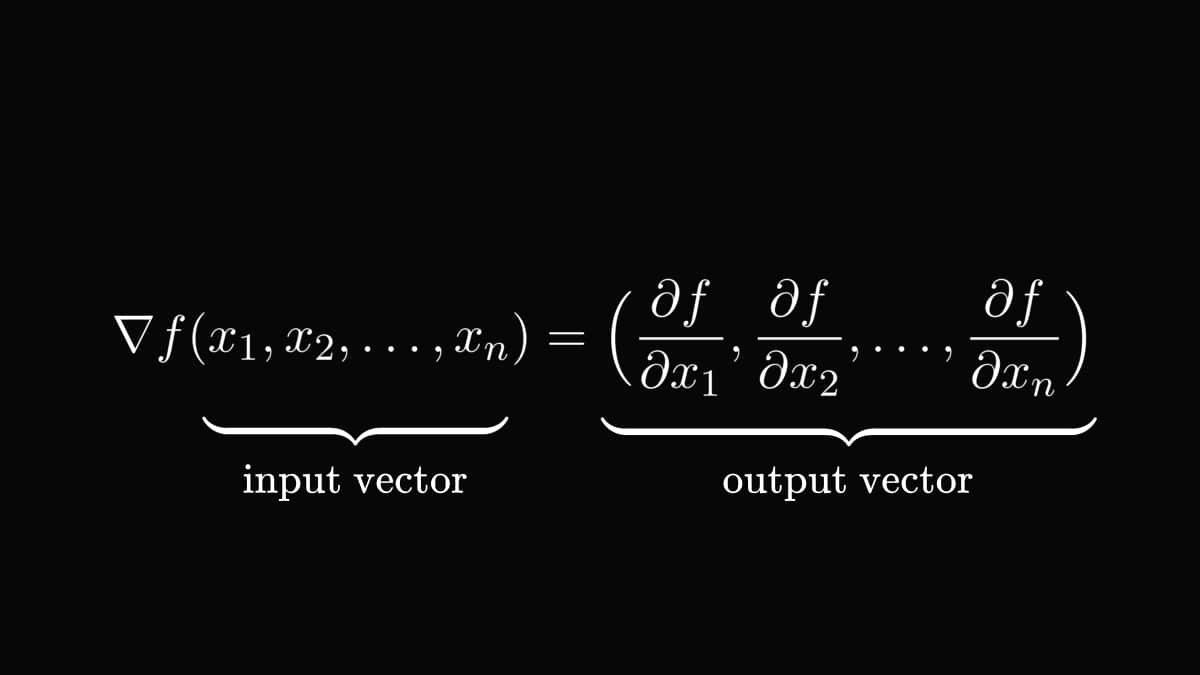We call this a gradient field. Let's visualize an example! This is how the vector field given by the gradient of $f(x, y) = x^2 + y^2$ looks.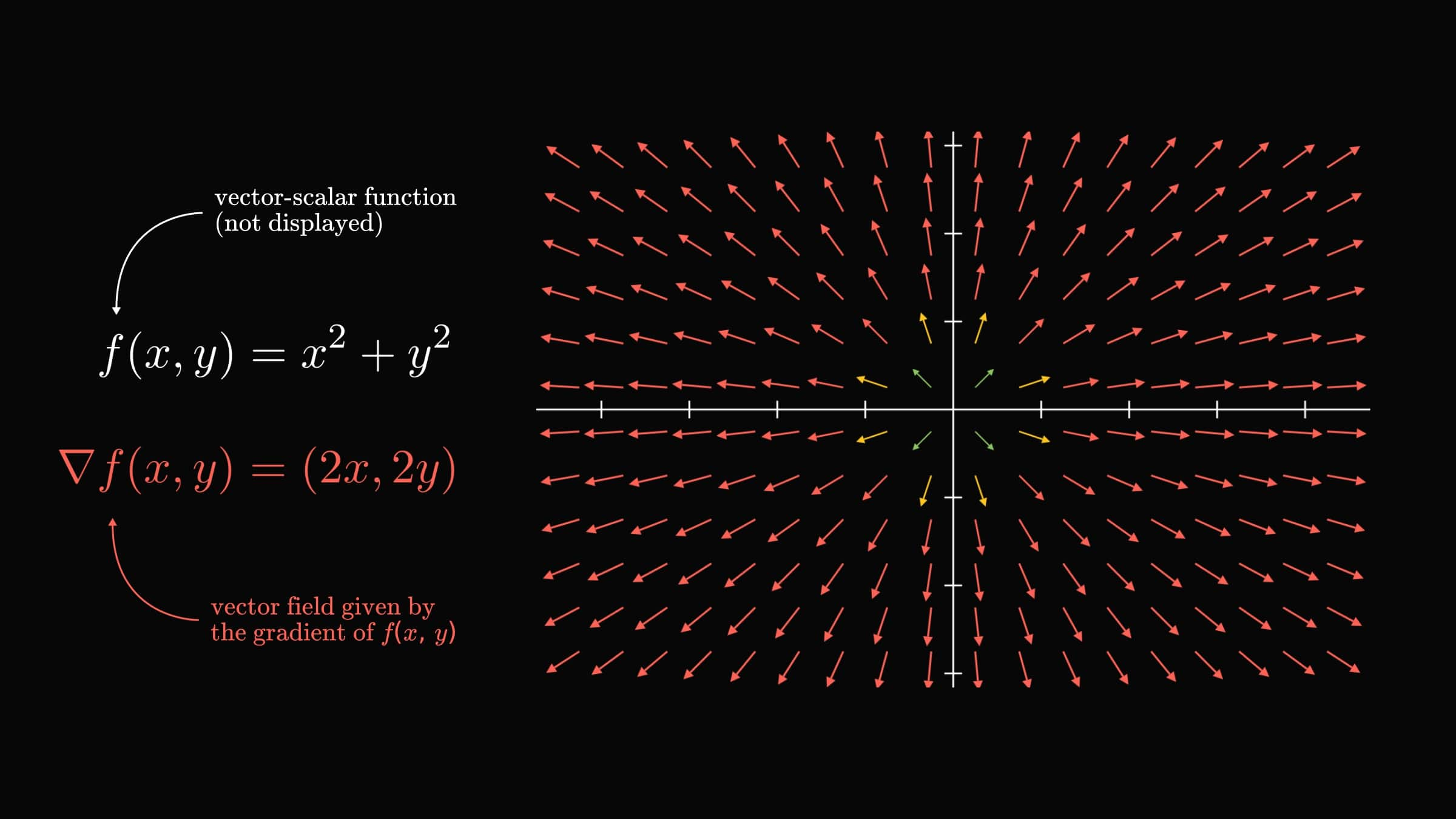It is important to note that not all vector-vector functions are gradient fields! For instance, $f(x, y) = (x - xy, xy - y)$ cannot be a gradient.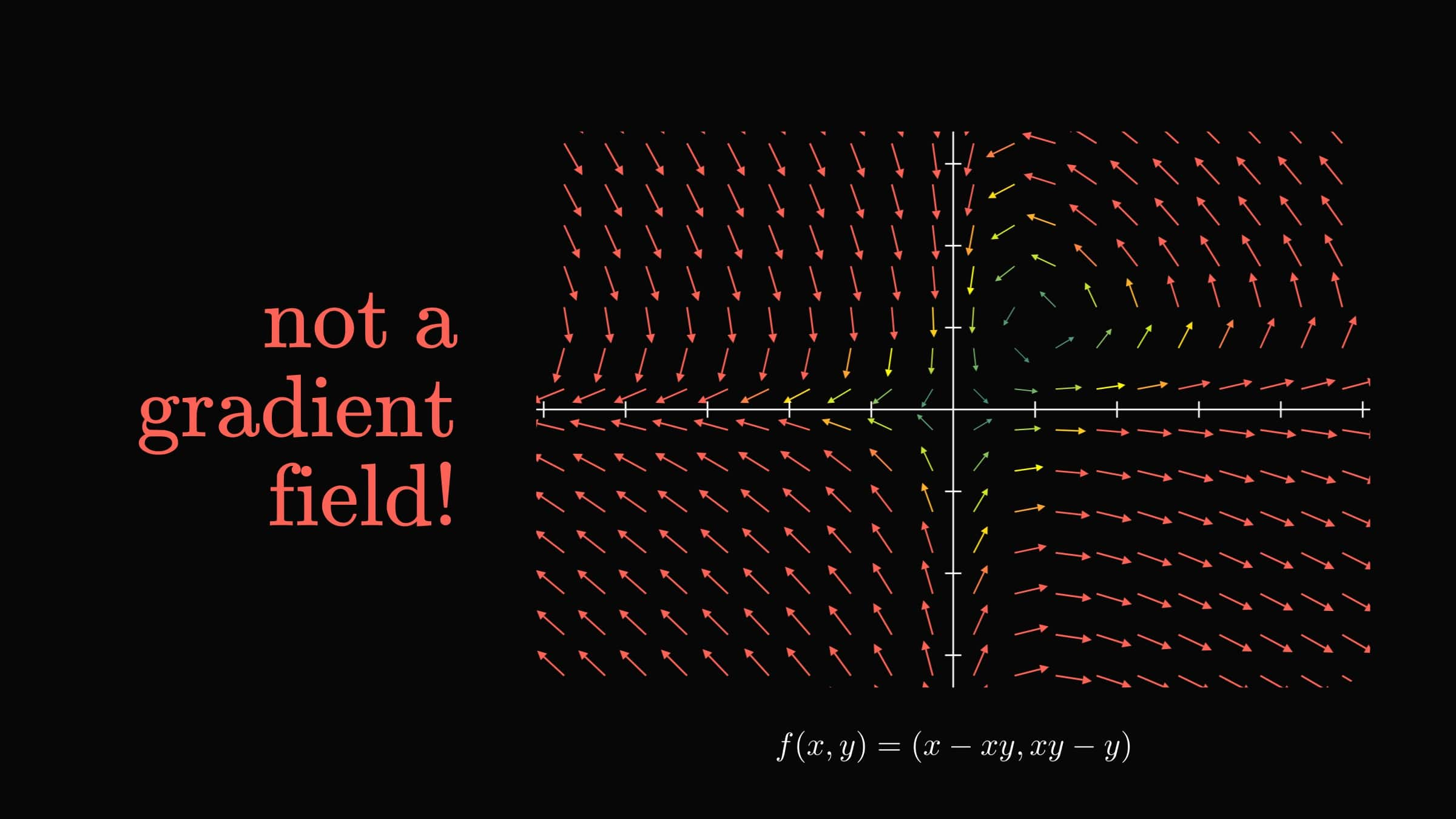Can you figure out the reason why? (Hint: take a look at the partial derivatives of $f(x, y)$.)

Next up, we have scalar-vector functions, that is, curves.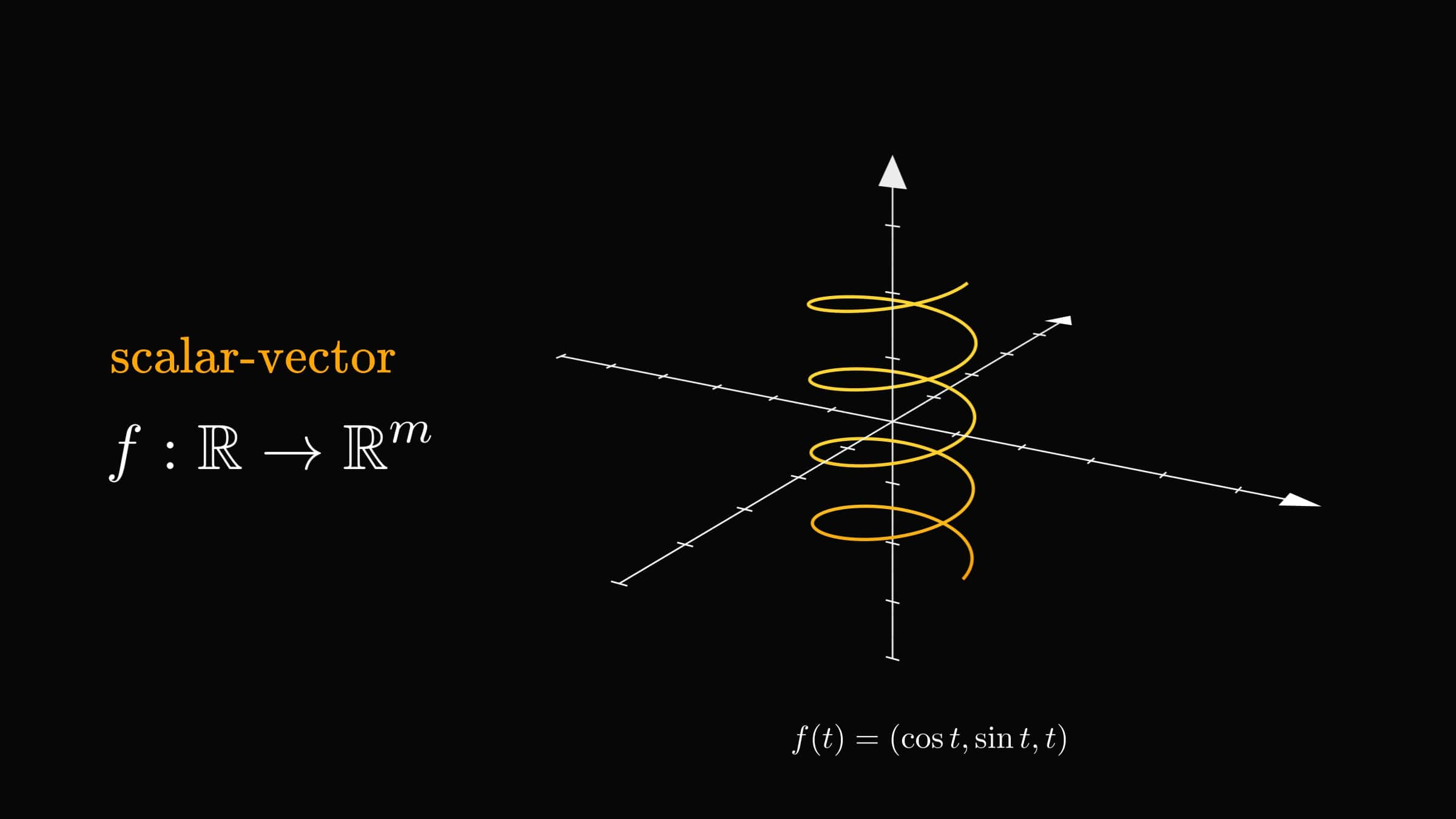Think about the scalar-vector function $f(t)$ as the trajectory of a particle at time $t$.

Technically, there is only a single variable involved. Yet, curves play an essential role in multivariable calculus.

Remember how vector-vector functions define force fields? Scalar-vector functions describe the trajectories of particles moving through them.